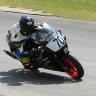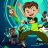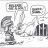# Opening Range every N Minutes#### korygill

##### Active member
VIP
Warehouse
This study displays an Opening Range (OR) on a chart every N minutes. It can be configured to display only during trading hours, or all hours. The code has only been tested on 3-day 1-minute charts for 30 and 60 minute OR bars. The full usefulness of this study is unknown and is left to the user. There are many times during the day where an Opening Range can provide insight into market action, and perhaps this will help users discover these.thinkScript Code

Rich (BB code):
``````# Orbit
#
# Description
# Draws the Opening Range (OR) for each recurring time period.
# Suggested use on 3d/1m chart with 30 min or 60 min ranges.
#
# Author: Kory Gill, @korygill
#
# Comment out unnecessary portions to preserve TOS memory and enhance speed
#

input timeOffsetFromEST = -300; #hint timeOffsetFromEST: 24-hour time offset from EST (-300 for PST)

input frequencyInMinutes = 60; #hint frequencyInMinutes: 30 mins or 60 mins recommended

input showAllMarketHours = no; #hint showAllMarketHours: if yes, all hours highlighted, not just market hours

# Common variables. Using variables reduces calls to TOS iData server.

# iData Definitions
def vHigh = high;
#def initHigh =  CompoundValue(1, high, high);  # creates an initialized variable for high
def vLow = low;
#def initLow = CompoundValue(1, low, low);
#def vOpen = open;
#def initOpen = CompoundValue(1, open, open);
#def vClose = close;
#def initClose = CompoundValue(1, close, close);
#def vVolume = volume;
#def initVolume = CompoundValue(1, volume, volume);
def nan = Double.NaN;

# Bar Time & Date
#def bn = BarNumber();
#def currentBar = HighestAll(if !IsNaN(vHigh) then bn else nan);
#def Today = GetDay() ==GetLastDay();
#def time = GetTime();
#def GlobeX = GetTime() < RegularTradingStart(GetYYYYMMDD());
#def globeX_v2 = if time crosses below RegularTradingEnd(GetYYYYMMDD()) then bn else GlobeX;
#def RTH = GetTime() > RegularTradingStart(GetYYYYMMDD());
#def RTH_v2 = if time crosses above RegularTradingStart(GetYYYYMMDD()) then bn else RTH;

# Bars that start and end the sessions
#def rthStartBar    = CompoundValue(1,
#                         if   !IsNaN(vClose)
#                         &&   time crosses above RegularTradingStart(GetYYYYMMDD())
#                         then bn
#                         else rthStartBar, 0);
#def rthEndBar      = CompoundValue(1,
#                         if   !IsNaN(vClose)
#                         &&   time crosses above RegularTradingEnd(GetYYYYMMDD())
#                         then bn
#                         else rthEndBar, 1);
#def globexStartBar = CompoundValue(1,
#                         if   !IsNaN(vClose)
#                         &&   time crosses below RegularTradingEnd(GetYYYYMMDD())
#                         then bn
#                         else globexStartBar, 1);
#def rthSession = if bn crosses above rthStartBar #+ barsExtendedBeyondSession
#                    then 1
#                    else if   bn crosses above rthEndBar #+ barsExtendedBeyondSession
#                         then 0
#                    else rthSession;

#
# Settings for well-known symbols
#
def startTime;

if (GetSymbol() == "/ES:XCME" or GetSymbol() == "/MES:XCME") {
startTime = 0630 - timeOffsetFromEST;
}
else if (GetSymbol() == "/NQ:XCME" or GetSymbol() == "/MNQ:XCME") {
startTime = 0630 - timeOffsetFromEST;
}
else if (GetSymbol() == "/YM:XCBT" or GetSymbol() == "/MYM:XCBT") {
startTime = 0630 - timeOffsetFromEST;
}
else if (GetSymbol() == "/RTY:XCME" or GetSymbol() == "/M2K:XCME") {
startTime = 0630 - timeOffsetFromEST;
}
else if (GetSymbol() == "/CL:XNYM") {
startTime = 0600 - timeOffsetFromEST;
}
else if (GetSymbol() == "/QM:XNYM") {
startTime = 0600 - timeOffsetFromEST;
}
else if (GetSymbol() == "/GC:XCEC") {
startTime = 0520 - timeOffsetFromEST;
}
else if (GetSymbol() == "/SI:XCEC") {
startTime = 0525 - timeOffsetFromEST;
}
else if (GetSymbol() == "/6J:XCME") {
startTime = 0520 - timeOffsetFromEST;
# there is a bug somewhere using 1200 I saw once.
# if you have issues, use 1159 below.
}
else {
# have to have an else...guess
startTime = 0630 - timeOffsetFromEST;
}

def modMinutes = (SecondsFromTime(startTime) % (frequencyInMinutes*60)) / 60;
#plot cm = modMinutes;

def showBar =  modMinutes != (frequencyInMinutes - 1) and  modMinutes != -1;
#plot sb = showBar;

def orbitActive = if showAllMarketHours or
SecondsFromTime(startTime) >= 0)
then 1
else 0;

def orbitHigh = if orbitActive and modMinutes == 0
then vHigh
else orbitHigh;

def orbitLow = if orbitActive and modMinutes == 0
then vLow
else orbitLow;

plot pOrbitHigh = if orbitActive and showBar and orbitHigh != 0 then orbitHigh else nan;
plot pOrbitLow = if orbitActive and showBar and orbitLow != 0 then orbitLow else nan;

pOrbitHigh.SetDefaultColor(Color.WHITE);
pOrbitLow.SetDefaultColor(Color.WHITE);

#def orbitWidth = orbitHigh - orbitLow;
#plot pOrbitWidth = if orbitActive and showBar and orbitWidth != 0 then orbitWidth else nan;
#pOrbitWidth.Hide();

http://tos.mx/TKC4oP

Last edited:
•skynetgenIndicators 0Custom 71Indicators 4Indicators 0